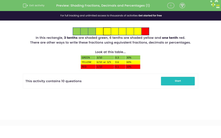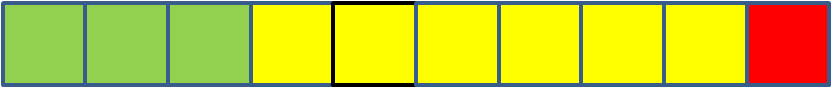# Shading Fractions, Decimals and Percentages (1)

In this worksheet, students identify the fraction, decimal or percentage shaded in a rectangle.Key stage:  KS 2

Curriculum topic:   Number: Fractions, Decimals and Percentages

Curriculum subtopic:   Know Fraction-Decimal-Percentage Equivalents

Difficulty level:#### Worksheet OverviewIn this rectangle, 3 tenths are shaded green, 6 tenths are shaded yellow and one tenth red.

There are other ways to write these fractions using equivalent fractions, decimals or percentages.

Look at this table...If we remember that the columns after the decimal point are tenths, hundredths and thousandths, this will help us convert fractions to decimals:

2/10 = 0.2

5/100 = 0.05

8/1000 = 0.008

Percentage is out of 100:

3/10 = 30/100 = 30%

0.7 = 7/10 = 70/100 = 70%

### What is EdPlace?

We're your National Curriculum aligned online education content provider helping each child succeed in English, maths and science from year 1 to GCSE. With an EdPlace account you’ll be able to track and measure progress, helping each child achieve their best. We build confidence and attainment by personalising each child’s learning at a level that suits them.

Get started